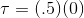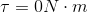# SAT II Physics : Other Mechanical Forces

## Example Questions

### Example Question #1 : Forces

A wrench is being used to tighten a bolt. The center of the bolt is the pivot point, and the wrench is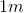long. If a force of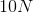is applied at the midpoint of the wrench and that force is parallel to the wrench, what is the torque?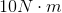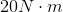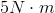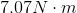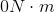Explanation:

The equation for torque is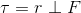The force is applied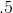meters from the pivot. and in this case because the force is parallel, the perpendicular component of force is zero, so: